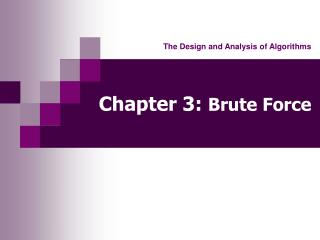Download PresentationChapter 3: Brute Force

# Chapter 3: Brute Force

Download Presentation## Chapter 3: Brute Force

- - - - - - - - - - - - - - - - - - - - - - - - - - - E N D - - - - - - - - - - - - - - - - - - - - - - - - - - -
##### Presentation Transcript

1. The Design and Analysis of Algorithms Chapter 3:Brute Force

2. Chapter 3. Brute Force Algorithms • Basic Idea • Brute Force Search and Sort • Brute Force String Matching • Closest Pair and Convex Hull Problems • Exhaustive Search • Conclusion

3. Basic Idea • A straightforward approach to solve a problem based on the problem’s statement and definitions of the concepts involved. • Example Computing anbased on the definition of exponentiation: an = a* a* a* …. * a (a > 0, n a nonnegative integer)

4. Brute Force Search and Sort • Sequential Search O(n) • Selection Sort O(n2) • Bubble Sort O(n2)

5. Brute Force String Matching Pattern: program Text: Write a program. program program … program Comparisons: (n*m) in the worst possible case (n+m) (n) in the average case.

6. Closest Pair Problem Find the two closest points in a set of n points in k-dimensional space. Algorithm ClosestPairPoints(P) dmin ← ∞ for i ← 1 to n-1 do for j ← i + 1 to n do d ← sqrt ((xi – xj) 2 + (yi – yj)2) if d < dmin dmin ← d (n2)

7. Convex Hull Problem • Convex set: For any two points P and Q in the set, the entire line segment with the end points at P and Q belongs to the set • Convex hull of a set S of points is the smallest convex set containing S The convex hull of any set S of n > 2 points is a convex polygon with the vertexes at some of the points of S.

8. Convex Hull Problem Algorithm: for each of n(n-1)/2 pairs of distinct points for each of the other n – 2 points find the sign of ax + by – c The time efficiency of this algorithm is O(n3).

9. Exhaustive Search • State-space search • Given an initial state, • a goal state, and • a set of operations, • find a sequence of operations that transforms the initial state to the goal state. • The solution process can be represented as a tree

10. Exhaustive Search Combinatorial problems • Traveling Salesman – permutations  ((N-1)!) • Knapsack – subsets  (2N) • Assignment problem – permutations  (N!)

11. Conclusion - Strengths • Wide applicability, simplicity • Reasonable algorithms for some important problems such as searching, string matching, and matrix multiplication • Standard algorithms for simple computational tasks such as sum and product of n numbers, and finding maximum or minimum in a list

12. Conclusion - Weaknesses • Brute Force approach rarely yields efficient algorithms • Some brute force algorithms are unacceptably slow • Brute Force approach is neither as constructive nor creative as some other design techniques